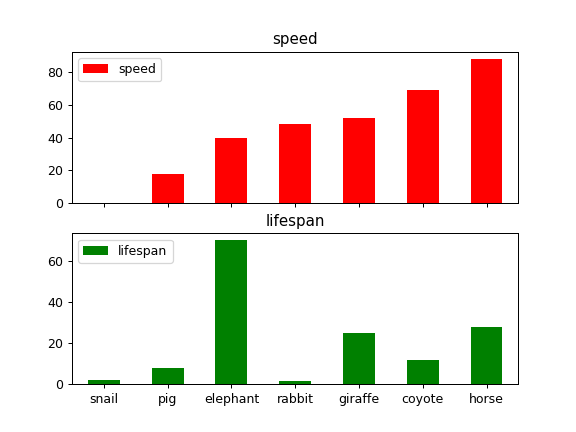# pandas.Series.plot.bar¶

Series.plot.bar(self, x=None, y=None, **kwargs)[source]

Vertical bar plot.

A bar plot is a plot that presents categorical data with rectangular bars with lengths proportional to the values that they represent. A bar plot shows comparisons among discrete categories. One axis of the plot shows the specific categories being compared, and the other axis represents a measured value.

Parameters
xlabel or position, optional

Allows plotting of one column versus another. If not specified, the index of the DataFrame is used.

ylabel or position, optional

Allows plotting of one column versus another. If not specified, all numerical columns are used.

**kwargs

Additional keyword arguments are documented in DataFrame.plot().

Returns
matplotlib.axes.Axes or np.ndarray of them

An ndarray is returned with one matplotlib.axes.Axes per column when subplots=True.

DataFrame.plot.barh

Horizontal bar plot.

DataFrame.plot

Make plots of a DataFrame.

matplotlib.pyplot.bar

Make a bar plot with matplotlib.

Examples

Basic plot.

>>> df = pd.DataFrame({'lab':['A', 'B', 'C'], 'val':[10, 30, 20]})
>>> ax = df.plot.bar(x='lab', y='val', rot=0)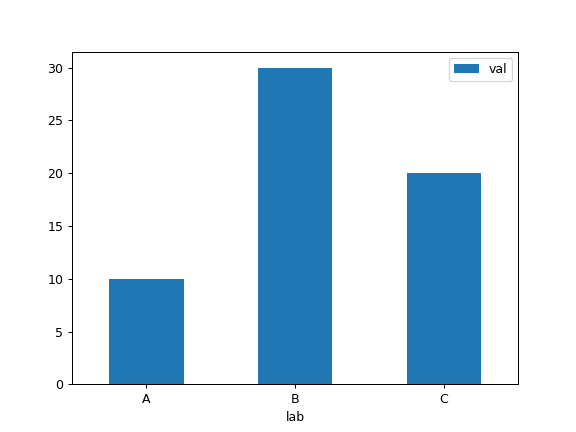Plot a whole dataframe to a bar plot. Each column is assigned a distinct color, and each row is nested in a group along the horizontal axis.

>>> speed = [0.1, 17.5, 40, 48, 52, 69, 88]
>>> lifespan = [2, 8, 70, 1.5, 25, 12, 28]
>>> index = ['snail', 'pig', 'elephant',
...          'rabbit', 'giraffe', 'coyote', 'horse']
>>> df = pd.DataFrame({'speed': speed,
...                    'lifespan': lifespan}, index=index)
>>> ax = df.plot.bar(rot=0)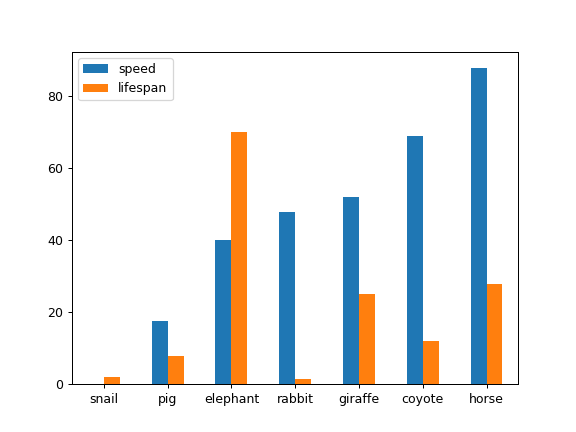Instead of nesting, the figure can be split by column with subplots=True. In this case, a numpy.ndarray of matplotlib.axes.Axes are returned.

>>> axes = df.plot.bar(rot=0, subplots=True)
>>> axes.legend(loc=2)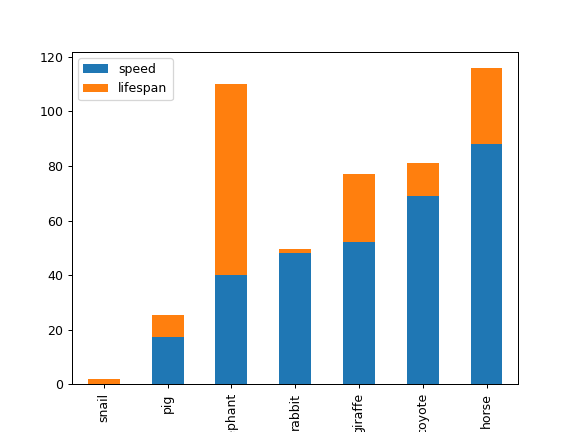Plot a single column.

>>> ax = df.plot.bar(y='speed', rot=0)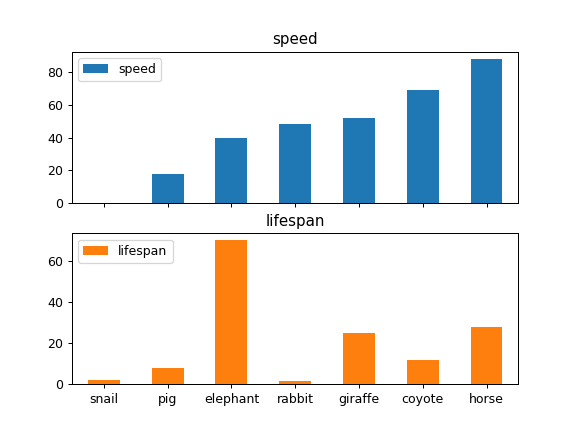Plot only selected categories for the DataFrame.

>>> ax = df.plot.bar(x='lifespan', rot=0)